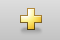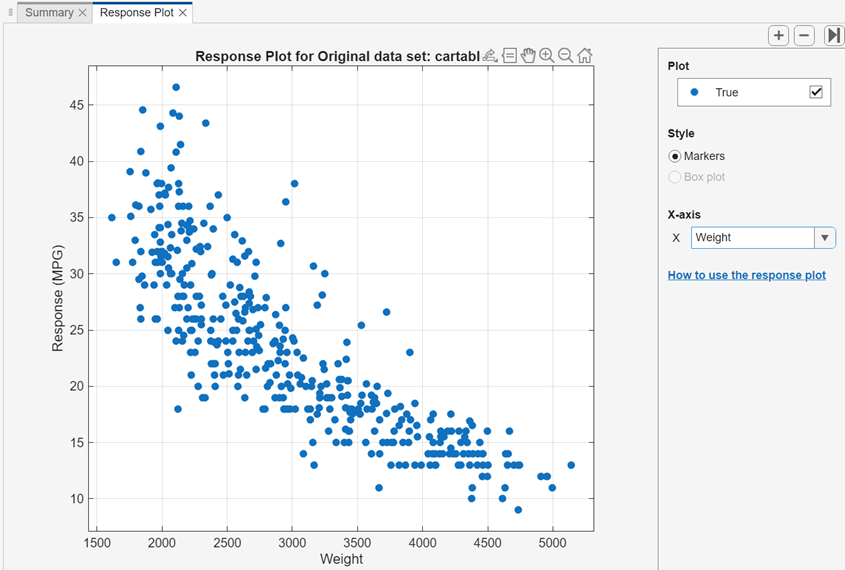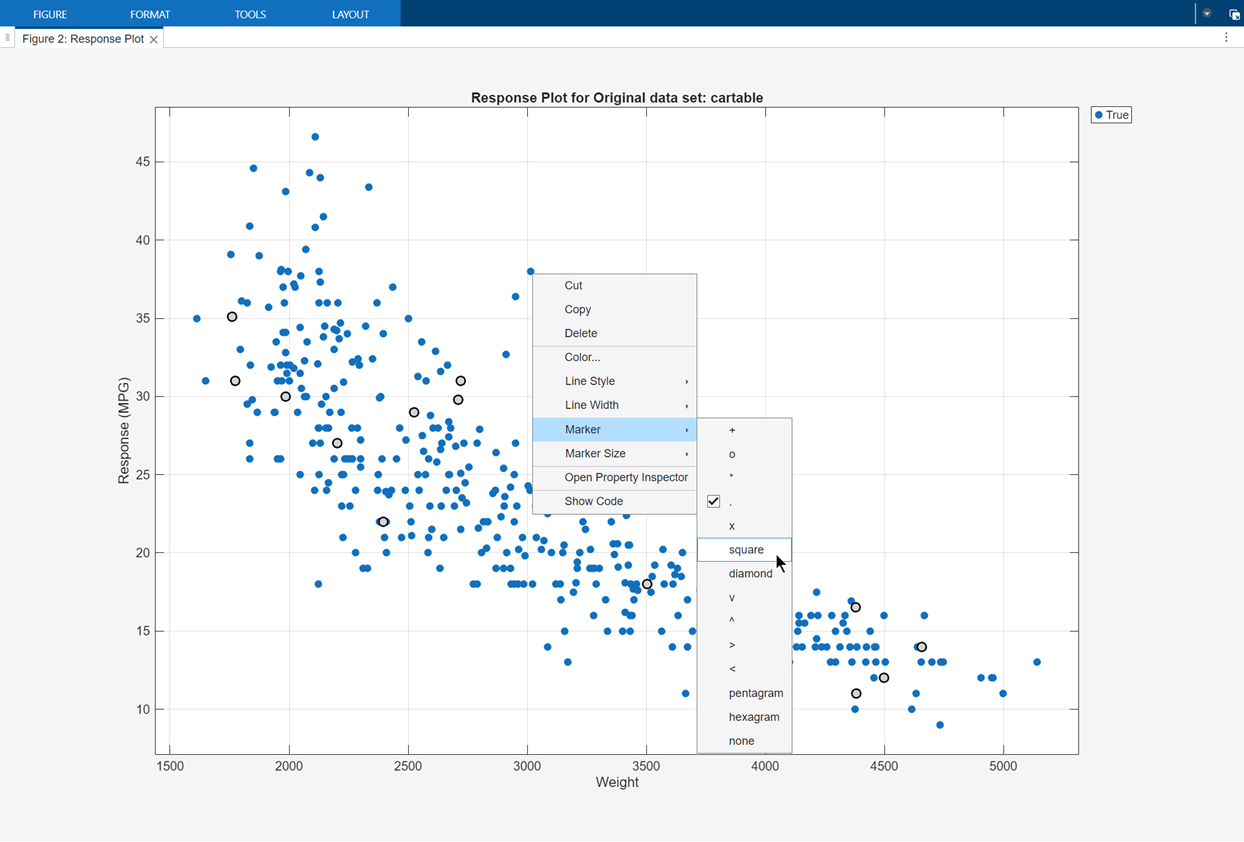## Export Plots in Regression Learner App

After you create plots interactively in the Regression Learner app, you can export your app plots to MATLAB® figures. You can then copy, save, or customize the new figures. Choose among the available plots: response plot, Predicted vs. Actual plot, residuals plot, minimum MSE plot, LIME explanations plot, Shapley explanations plot, and partial dependence plot.

• Before exporting a plot, make sure the plot in the app displays the same data that you want in the new figure.

• On the Learn, Test, or Explain tab, in the Export section, click . The app creates a figure from the selected plot.

• The new figure might not have the same interactivity options as the plot in the Regression Learner app.

• Additionally, the figure might have a different axes toolbar than the one in the app plot. For plots in Regression Learner, an axes toolbar appears above the top right of the plot. The buttons available on the toolbar depend on the contents of the plot. The toolbar can include buttons to export the plot as an image, add data tips, pan or zoom the data, and restore the view.• Copy, save, or customize the new figure, which is displayed in the figure window.

• To copy the figure, select Edit > Copy Figure. For more information, see Copy Figure to Clipboard from Edit Menu.

• To save the figure, select File > Save As. Alternatively, you can follow the workflow described in Customize Figure Before Saving.

• To customize the figure, click the Edit Plot buttonon the figure toolbar. Right-click the section of the plot that you want to edit. You can change the listed properties, which might include Color, Font, Line Style, and other properties. Or, you can use the Property Inspector to change the figure properties.

As an example, export a response plot in the app to a figure, customize the figure, and save the modified figure.

1. In the MATLAB Command Window, load the `carbig` data set.

```load carbig cartable = table(Acceleration,Cylinders,Displacement, ... Horsepower,Model_Year,Weight,Origin,MPG);```
2. Click the Apps tab.

3. In the Apps section, click the arrow to open the gallery. Under Machine Learning and Deep Learning, click Regression Learner.

4. On the Learn tab, in the File section, click.

5. In the New Session from Workspace dialog box, select the table `cartable` from the Data Set Variable list.

6. Click . Regression Learner creates a response plot of the data by default.

7. Change the x-axis data in the response plot to `Weight`.8. On the Learn tab, in the Export section, click .

9. In the new figure, click the Edit Plot buttonon the figure toolbar. Right-click the points in the plot. In the context menu, select Marker > square.10. To save the figure, select File > Save As. Specify the saved file location, name, and type.